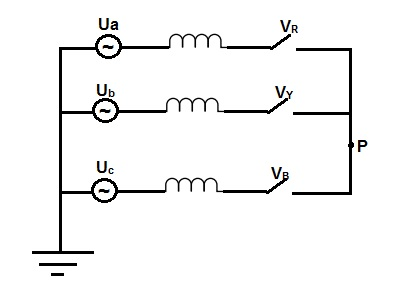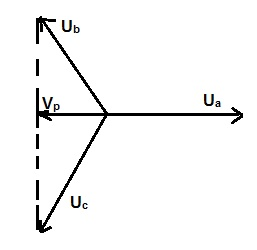# First Pole to Clear Factor- Calculation & Significance

First Pole to Clear Factor of a Circuit Breaker is the ratio of power frequency recovery voltage across the first pole to clear the arc to the normal phase to ground voltage when all the three poles of breaker are open. It is denoted as kpp. If Vcb be the recovery voltage across the first pole of CB and Vph be the phase to ground voltage then

First Pole to Clear Factor = Vcb / Vph

The value of kpp depends on the system grounding. For system with non-effectively neutral earthing, kpp = 1.5 while for system with effectively neutral earthing, kpp = 1.3

Let us now understand the concept of first pole to clear factor in detail. We know that a circuit breaker is used to make or break circuit or part of circuit in power system. Since the power system is mostly inductive in nature, therefore the current flowing in the network is lags the voltage. This definitely means that whenever the voltage is zero, current is at its peak and when voltage is at its peak, current is zero. In circuit breaker, the basic philosophy used to break the circuit current is to open the breaker contacts at natural current zero. But the three phases current are separated from each other by an angle of 120 degree. This means that the natural zero of the three phases will take place at three different points of time. Figure below shows the current interruption for an assumed fault.As clear from the figure, the current of Y phase first comes to zero at 53.5 ms followed by R and B phases (phase current first coming to natural zero is dependent on the time of occurrence of fault). This means that first Y pole will be opened and then R and B pole of breaker. When Y poles opens in response to any fault, the remaining two poles are closed. This gives rise to high recovery voltage across the breaker contacts when compared with phase to ground voltage when all the three poles are open.

Let us consider a three phase fault as shown in figure below. The figure shows the simplified equivalent circuit of effectively earthed neutral with three phase fault without involving earth. When the very first pole (here it is assumed that R pole opens first) interrupts the fault, the symmetry of the system is lost and the system goes into a pure a phase to phase fault condition. Due to this, the voltage of short circuit point shifts.The power frequency recovery voltage across the first opening pole i.e. R pole (assumed) is nothing but the difference between the source side voltage and network side voltage. In our case, this will be different between the source side voltage Ua and network side voltage Vp (voltage at short circuit point). Thus,

VR =   Recovery Voltage across the first opening pole

= Ua – Vp   …………..(1)

From the symmetry of the system when the system goes into pure phase to phase fault, the voltage at the short circuit point will be average of the Ub and Uc. The phasor diagram is shown below.Vp = (phasor sum of Ub and Uc) / 2

= 0.5Ub

But magnitude of Ua, Ub and Uc are same, hence

Vp = 0.5Ua

Therefore from (1),

VR = Ua + 0.5Ua

= 1.5Ua

Hence from the definition,

First Pole to Clear Factor, kpp = 1.5Ua / Ua

= 1.5

Thus we see that, value of first pole to clear factor for effectively gounded neutral system with ungrounded fault is 1.5.

For three phase to earth faults with effectively earth neutral, the value of kpp depend on the positive sequence and zero sequence reactance. The value of kpp for such condition is given as

kpp = 3 / [(X1 / X0) + 2]

where X1 = Positive Sequence Reactance

X0 = Zero Sequence Reactance

For grounded system as is the case with transmission line, the ratio of (X1 / X0) is 1/3 and therefore the value of first pole to clear factor is 1.3.

Significance of First Pole to Clear Factor:

First Pole to Clear Factor is basically the impact of system grounding on the Transient Recovery Voltage (TRV). Apart from system grounding, TRV depends on many other factors. All the three poles of circuit breaker can never open simultaneously; therefore first pole to clear factor must be taken care for the design of breaker. The dielectric strength of breaker must be sufficient enough to sustain the TRV across the first pole to clear the fault.

This site uses Akismet to reduce spam. Learn how your comment data is processed.